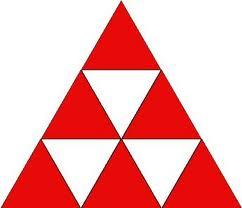# Calculate 4422

Calculate the perimeter of the triangle ABC if a = 12 cm, the angle beta is 38 degrees, and the gamma is 92 degrees.

o =  37.2996 cm

### Step-by-step explanation:

Try calculation via our triangle calculator.Did you find an error or inaccuracy? Feel free to write us. Thank you!

Tips for related online calculators
Check out our ratio calculator.